# Algebra 1 : How to find the nth term of an arithmetic sequence

## Example Questions

2 Next →

### Example Question #11 : How To Find The Nth Term Of An Arithmetic Sequence

The second term of an arithmetic sequence is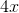; the fourth term is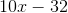. What is the first term?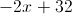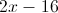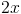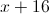Explanation:

The common difference between the terms is half that between the second and fourth terms - that is: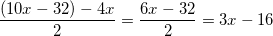Subtract this common difference from the second term to get the first: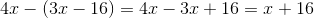### Example Question #21 : Sequences

An arithmetic sequence is given by the formula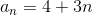.  What is the difference between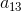and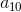Explanation:

You can either calculate the vaules ofandand subtract, or notice from the formula that each succesive number in the sequence is 3 larger than the previous

### Example Question #12 : How To Find The Nth Term Of An Arithmetic Sequence

Consider the following arithmetic sequence: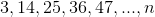What is the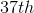term?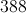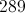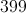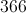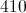Explanation:

A simple way to find the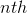term of an arithmetic sequence is to use the formula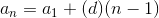.

Here,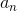is the term you are trying to find,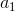is the first term, andis the common difference. For this question, the common difference is.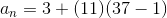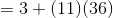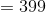### Example Question #12 : How To Find The Nth Term Of An Arithmetic Sequence

What is the 20th term of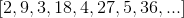?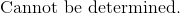Explanation:

Notice that every odd term is increasing at an increment of, since the numbers are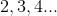.  We will ignore these terms and concentrate on only the even terms.

The even terms are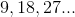for the second, fourth, and sixth terms respectively, which means that the numbers are a multiple of nine.

The equation that represents the terms for this sequence is: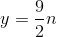, foreven terms since it only applies to the even terms that are divisible by nine and occurs after every two terms.

Substitute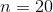to determine the 20th term.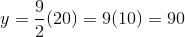The answer is:### Example Question #31 : Sequences

What is the 11th term in the following sequence?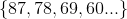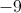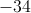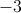Explanation:

First we need to show how this sequence is changing. Let's call the first number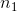, and the second number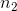, and so on.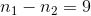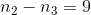Ok, so we have established that the sequence is shrinking by 9 each time. So now we need to calculate out to the 11th term. Starting from the first term, we need to subtract 9 ten times to get to the 11th term. So that would look like this.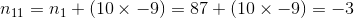2 Next →

### All Algebra 1 Resources